## Monday, November 21, 2011

### 3D Analogue to the Trapezoid (part 2): Truncated Pyramid

Since I wrote the post entitled 3D Analogue to the Trapezoid, I have learned that people already have something in mind when they type in terms to this effect. They are interested in a truncated pyramid. In the aforementioned post, I described a shape, namely, an irregular triangular prism, that was analogous to a trapezoid in terms of its volume formula (the proof is located here). Now, we look at a shape that is analogous to a trapezoid in terms of its appearance, but has an only loosely analogous volume formula.

The first observation we might make is that pyramids are really not very different from cones from a volume perspective. The simple volume formula for both is given by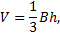where B is the area of the base and h is the height of the cone. This is true regardless of the shape of the base. The only requirement is that the same shape must continue all the way to the peak. In more technical language, the cross-section of the pyramid at distance b below the peak must be similar to the base and the corresponding linear dimensions must be in the ratio b:h. So, given a dimension of length d in the base, the corresponding dimension in the cross-section at b below the peak must have length d(b/h). This is part of the definition of a pyramid. At this point, a well-known, fancy theorem may be in order.

Theorem. Let A and B be similar (2-dimensional) shapes. Then,
Corresponding linear dimensions have a ratio of a:b if and only if the areas of A and B (or corresponding subareas) are in the ratio a2:b2.

In theorem form, this might seem like it’s from outer space, but notice how simply that works out with squares and circles. For example, if one square has a side of a and another of side b, then their areas are a2 and b2, respectively. The converse is obviously true as well, and it is the converse we will need.

Suppose we take the area of the top surface to be B1 and the area of the bottom surface to be B2. Also, take a to be the height of the part of the pyramid that has been removed and h to be the height of the truncated pyramid. Here’s a front orthographic view of the pyramid with the cut off part added back to the top: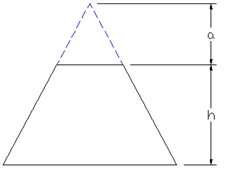Our theorem then tells us that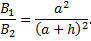If we solve for a, we get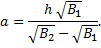The volume of our truncated pyramid is simply the volume of the full pyramid minus the volume of the part that has been cut off (truncated off):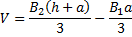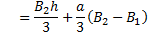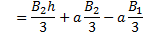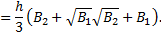It is noteworthy that we could have calculated a without calculating the areas B1 or B2. But we would need to know the ratio between a pair of corresponding sides, say d1 and d2 (see diagram below).

We would calculate a according to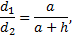which gives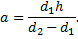Our volume formula will look a little nicer: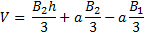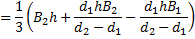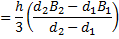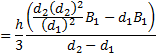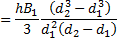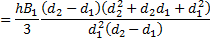Letting r = d2/d1, we get

The first volume formula makes somewhat of an analogy with the area formula of a trapezoid: We are taking the average (arithmetic mean) of
1. the bottom base area,
2. the top base area, and
3. the geometric mean of the top and bottom areas
and multiplying by the perpendicular distance between the top and bottom areas. I must say, that’s a closer analogy than I expected before I wrote this article.